# Selina Solutions Concise Mathematics Class 6 Chapter 9: Playing With Numbers Exercise 9(B)

Selina Solutions Concise Mathematics Class 6 Chapter 9 Playing With Numbers Exercise 9(B) provides a basic method in solving difficult questions with ease that are important for the exam. Students, who want to improve their problem-solving skills, are advised to practice Selina Solutions on a daily basis. The solutions are created by BYJU’S experts in order to help students to overcome exam fear. Those who aspire to score high marks and achieve success in the examination are suggested to refer to the solutions while revising the textbook questions. Students can download Selina Solutions Concise Mathematics Class 6 Chapter 9 Playing With Numbers Exercise 9(B), from the provided links below

## Selina Solutions Concise Mathematics Class 6 Chapter 9: Playing With Numbers Exercise 9(B) Download PDF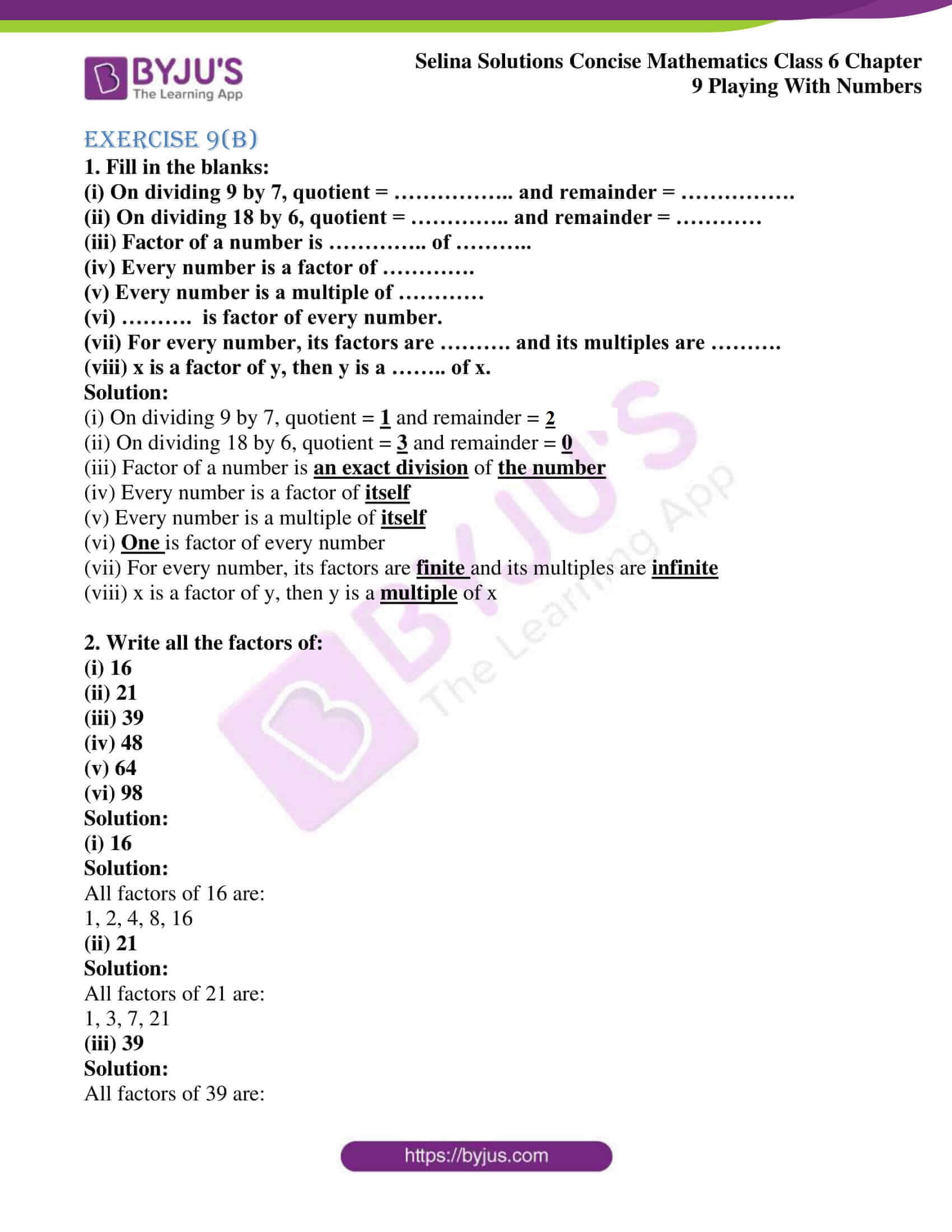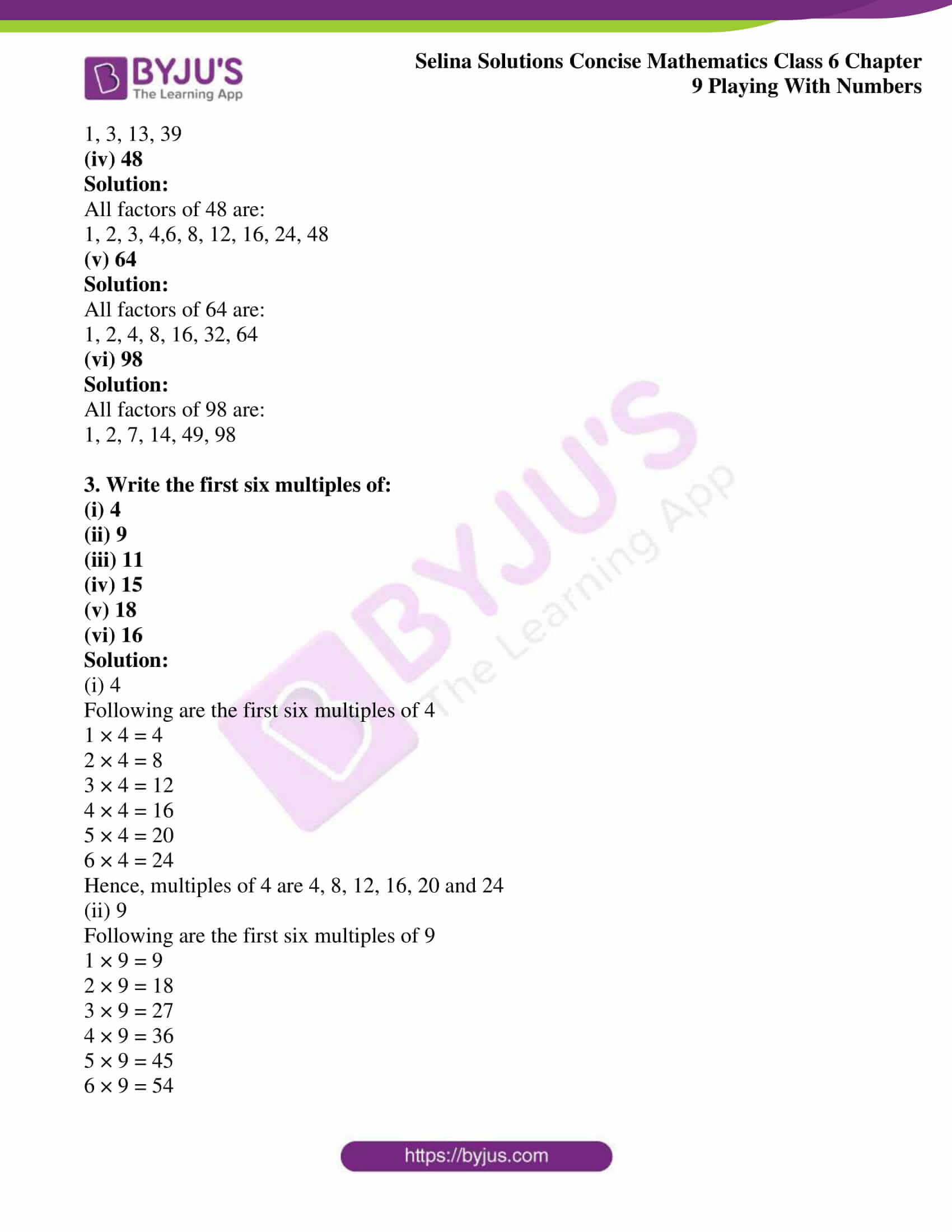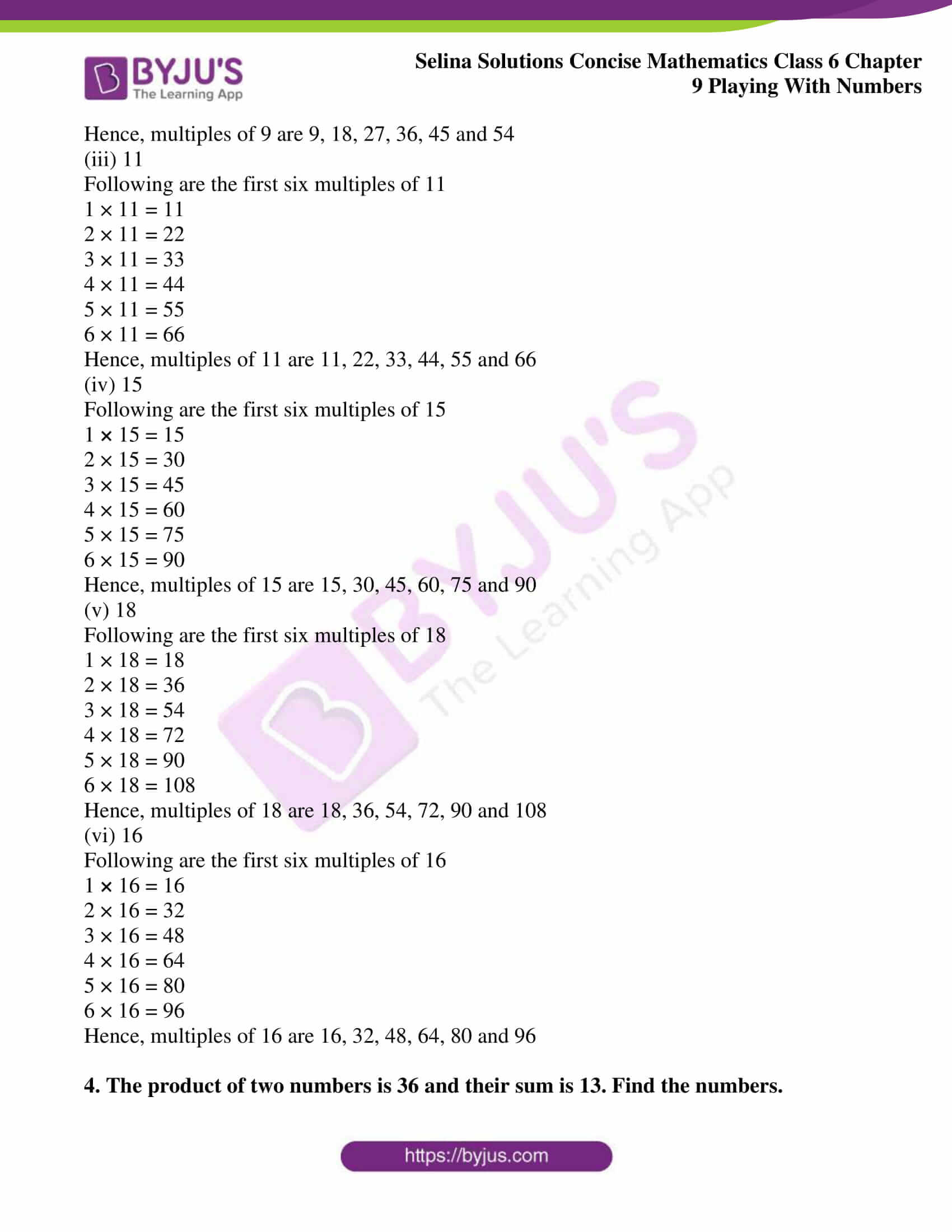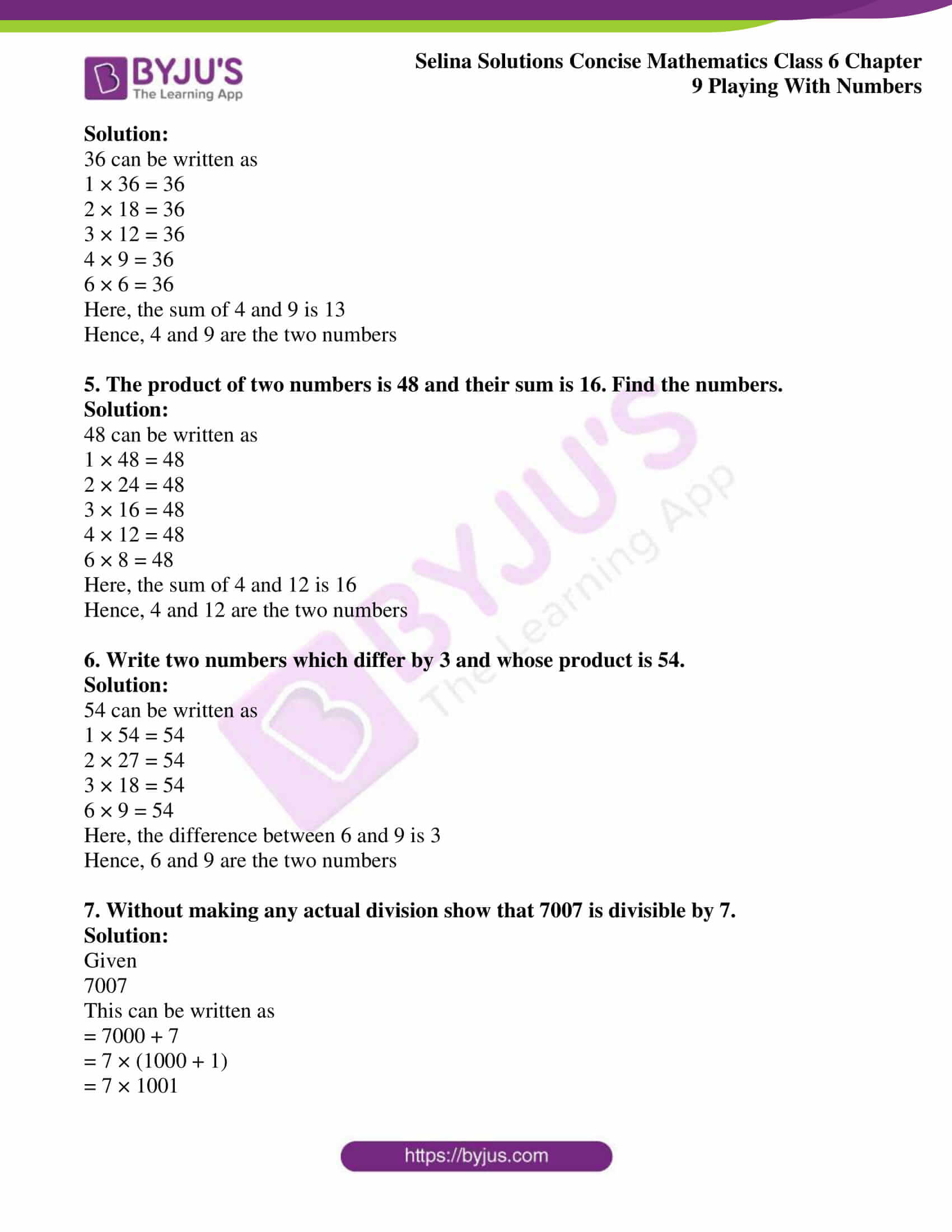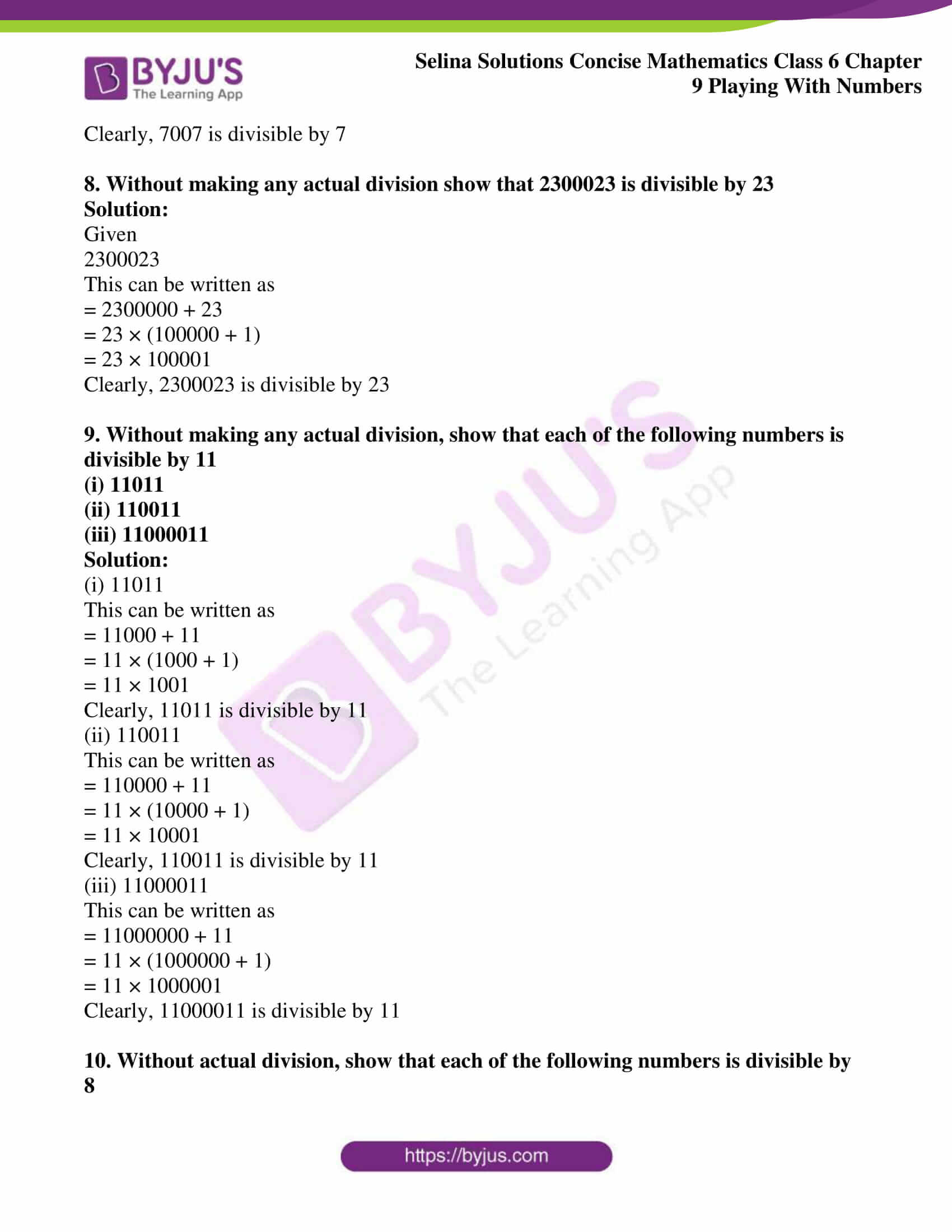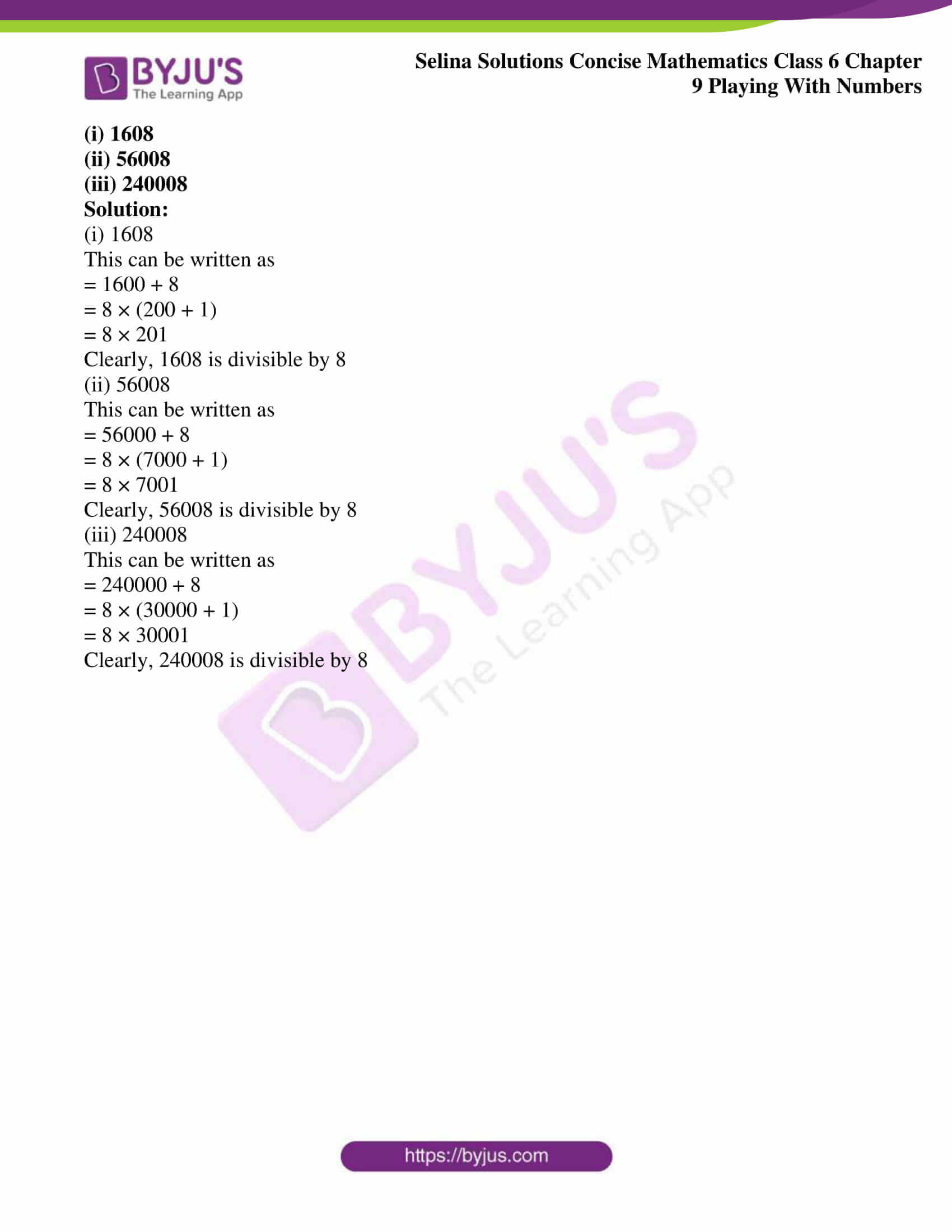### Access other exercises of Selina Solutions Concise Mathematics Class 6 Chapter 9 Playing With Numbers

Exercise 9(A) Solutions

Exercise 9(C) Solutions

### Access Selina Solutions Concise Mathematics Class 6 Chapter 9 Playing With Numbers Exercise 9(B)

#### Exercise 9(B)

1. Fill in the blanks:

(i) On dividing 9 by 7, quotient = …………….. and remainder = …………….

(ii) On dividing 18 by 6, quotient = ………….. and remainder = …………

(iii) Factor of a number is ………….. of ………..

(iv) Every number is a factor of ………….

(v) Every number is a multiple of …………

(vi) ………. is factor of every number.

(vii) For every number, its factors are ………. and its multiples are ……….

(viii) x is a factor of y, then y is a …….. of x.

Solution:

(i) On dividing 9 by 7, quotient = 1 and remainder = 2

(ii) On dividing 18 by 6, quotient = 3 and remainder = 0

(iii) Factor of a number is an exact division of the number

(iv) Every number is a factor of itself

(v) Every number is a multiple of itself

(vi) One is factor of every number

(vii) For every number, its factors are finite and its multiples are infinite

(viii) x is a factor of y, then y is a multiple of x

2. Write all the factors of:

(i) 16

(ii) 21

(iii) 39

(iv) 48

(v) 64

(vi) 98

Solution:

(i) 16

Solution:

All factors of 16 are:

1, 2, 4, 8, 16

(ii) 21

Solution:

All factors of 21 are:

1, 3, 7, 21

(iii) 39

Solution:

All factors of 39 are:

1, 3, 13, 39

(iv) 48

Solution:

All factors of 48 are:

1, 2, 3, 4,6, 8, 12, 16, 24, 48

(v) 64

Solution:

All factors of 64 are:

1, 2, 4, 8, 16, 32, 64

(vi) 98

Solution:

All factors of 98 are:

1, 2, 7, 14, 49, 98

3. Write the first six multiples of:

(i) 4

(ii) 9

(iii) 11

(iv) 15

(v) 18

(vi) 16

Solution:

(i) 4

Following are the first six multiples of 4

1 × 4 = 4

2 × 4 = 8

3 × 4 = 12

4 × 4 = 16

5 × 4 = 20

6 × 4 = 24

Hence, multiples of 4 are 4, 8, 12, 16, 20 and 24

(ii) 9

Following are the first six multiples of 9

1 × 9 = 9

2 × 9 = 18

3 × 9 = 27

4 × 9 = 36

5 × 9 = 45

6 × 9 = 54

Hence, multiples of 9 are 9, 18, 27, 36, 45 and 54

(iii) 11

Following are the first six multiples of 11

1 × 11 = 11

2 × 11 = 22

3 × 11 = 33

4 × 11 = 44

5 × 11 = 55

6 × 11 = 66

Hence, multiples of 11 are 11, 22, 33, 44, 55 and 66

(iv) 15

Following are the first six multiples of 15

1 × 15 = 15

2 × 15 = 30

3 × 15 = 45

4 × 15 = 60

5 × 15 = 75

6 × 15 = 90

Hence, multiples of 15 are 15, 30, 45, 60, 75 and 90

(v) 18

Following are the first six multiples of 18

1 × 18 = 18

2 × 18 = 36

3 × 18 = 54

4 × 18 = 72

5 × 18 = 90

6 × 18 = 108

Hence, multiples of 18 are 18, 36, 54, 72, 90 and 108

(vi) 16

Following are the first six multiples of 16

1 × 16 = 16

2 × 16 = 32

3 × 16 = 48

4 × 16 = 64

5 × 16 = 80

6 × 16 = 96

Hence, multiples of 16 are 16, 32, 48, 64, 80 and 96

4. The product of two numbers is 36 and their sum is 13. Find the numbers.

Solution:

36 can be written as

1 × 36 = 36

2 × 18 = 36

3 × 12 = 36

4 × 9 = 36

6 × 6 = 36

Here, the sum of 4 and 9 is 13

Hence, 4 and 9 are the two numbers

5. The product of two numbers is 48 and their sum is 16. Find the numbers.

Solution:

48 can be written as

1 × 48 = 48

2 × 24 = 48

3 × 16 = 48

4 × 12 = 48

6 × 8 = 48

Here, the sum of 4 and 12 is 16

Hence, 4 and 12 are the two numbers

6. Write two numbers which differ by 3 and whose product is 54.

Solution:

54 can be written as

1 × 54 = 54

2 × 27 = 54

3 × 18 = 54

6 × 9 = 54

Here, the difference between 6 and 9 is 3

Hence, 6 and 9 are the two numbers

7. Without making any actual division show that 7007 is divisible by 7.

Solution:

Given

7007

This can be written as

= 7000 + 7

= 7 × (1000 + 1)

= 7 × 1001

Clearly, 7007 is divisible by 7

8. Without making any actual division show that 2300023 is divisible by 23

Solution:

Given

2300023

This can be written as

= 2300000 + 23

= 23 × (100000 + 1)

= 23 × 100001

Clearly, 2300023 is divisible by 23

9. Without making any actual division, show that each of the following numbers is divisible by 11

(i) 11011

(ii) 110011

(iii) 11000011

Solution:

(i) 11011

This can be written as

= 11000 + 11

= 11 × (1000 + 1)

= 11 × 1001

Clearly, 11011 is divisible by 11

(ii) 110011

This can be written as

= 110000 + 11

= 11 × (10000 + 1)

= 11 × 10001

Clearly, 110011 is divisible by 11

(iii) 11000011

This can be written as

= 11000000 + 11

= 11 × (1000000 + 1)

= 11 × 1000001

Clearly, 11000011 is divisible by 11

10. Without actual division, show that each of the following numbers is divisible by 8

(i) 1608

(ii) 56008

(iii) 240008

Solution:

(i) 1608

This can be written as

= 1600 + 8

= 8 × (200 + 1)

= 8 × 201

Clearly, 1608 is divisible by 8

(ii) 56008

This can be written as

= 56000 + 8

= 8 × (7000 + 1)

= 8 × 7001

Clearly, 56008 is divisible by 8

(iii) 240008

This can be written as

= 240000 + 8

= 8 × (30000 + 1)

= 8 × 30001

Clearly, 240008 is divisible by 8